# Shortest path

The shortest paths problem is one of the most fundamental problems in graph theory. Given a directed graph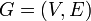$G = (V,E)$, possibly weighted, and a set of pairs of vertices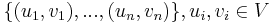$\{(u_1,v_1), ..., (u_n,v_n)\}, u_i, v_i \in V$, the problem is to compute, for each$i$, a path in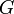$G$ from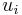$u_i$ to$v_i$ (a list of vertices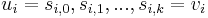$u_i = s_{i,0}, s_{i,1}, ..., s_{i,k} = v_i$ such that for all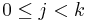$0 \leq j < k$,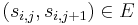$(s_{i,j},s_{i,j+1}) \in E$) such that no other path in$G$ from$u_i$ to$v_i$ has a lower total weight.

Shortest paths in undirected graphs can be computed by replacing each undirected edge with two arcs of the same weight, one going in each direction, to obtain a directed graph.

## Variations

Three variations of the shortest path algorithm exist, and they are discussed in the following sections.

• In the single-pair shortest path problem, there is only one pair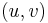$(u,v)$ in the problem set. In other words the shortest path is desired between a single pair of vertices.
• In the single-source shortest paths problem, the problem set is of the form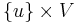$\{u\} \times V$. One vertex,$u$, is designated the source, and we wish to find the shortest paths from the source to all other vertices. (To solve the analogous single-destination shortest paths problem, we merely reverse the directions of all edges, which reduces it to single-source.)
• In the all-pairs shortest paths problem, the problem set is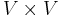$V \times V$; that is, we wish to know the shortest paths from every vertex to every other vertex.

## Approach

All the shortest paths algorithms discussed in this article have the same basic approach. At their core, they compute not the shortest paths themselves, but the distances. Using information computed in order to compute the distances, one can easily then reconstruct the paths themselves. They begin with the knowledge that the distance from any vertex to itself is zero, and they overestimate all other distances they need. (By this it is meant that they find a number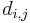$d_{i,j}$ for each pair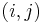$(i,j)$ under consideration such that the distance from$i$ to$j$ is less than or equal to$d_{i,j}$.) At some point, all overestimates will be refined, perhaps gradually, perhaps at once, so that once the algorithm has terminated, they are exactly the correct distances.

### Relaxation

There are theoretically many ways to refine overestimates but a specific way, known as relaxation, is used in all the algorithms discussed in this article. Relaxation can take place when three conditions are met:

1. The currently best overestimate for the distance from some vertex$i$ to some vertex$j$ is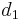$d_1$;
2. The currently best overestimate for the distance from$j$ to some vertex$k$ is$d_2$;
3. The currently best overestimate for the distance from$i$ to$k$ is greater than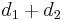$d_1+d_2$. (This includes the case in which it is infinite.)

Relaxation refines the best overestimate for the distance from$i$ to$k$ by setting it to$d_1+d_2$, which is better than its current value.